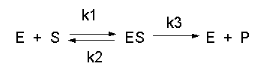# B4. Steady State Enzyme-Catalyzed ReactionsIn this derivation, we will consider the following equations and all the rate constants, and will not arbitrarily assume that $$k_2 \gg k_3$$. We will still assume that $$S \gg E_o$$ and that $$P_o = 0$$. An added assumption, however, is that dES/dt is approximately 0. Look at this assumption this way. When an excess of S is added to E, ES is formed. In the rapid equilibrium assumption, we assumed that it would fall back to $$E + S$$ faster than it would go onto product. In this case, we will assume that it might go on to product either less or more quickly than it will fall back to $$E + S$$.

In either case, a steady state concentration of $$ES$$ arises within a few milliseconds, whose concentration does not change significantly during the initial part of the reaction under which the initial rates are measured. Therefore, $$dES/dt$$ is about 0. In the rapid equilibrium derivation, we observed that $$v = k_3ES$$. We then solved for $$ES$$ using $$K_s$$ and mass balance of E. In the steady state assumption, the equation v = k3ES still holds, but know we will solve for ES using the steady state assumption that $$dES/dt =0$$.

$v = k_3[ES] \label{1}$

$\dfrac{d[ES]}{dt} = k_1[E][S] - k_2[ES] -k_3[ES] = 0 \label{6}$

$k_1[E][S] = (k_2 + k_3)[ES]$

$k_1(E_o-[ES])(S) = (k_2 + k_3)(ES)$

$k_1[E_o][S] - k_1[ES][S] = (k_2 + k_3)[ES]$

$k_1[E_o][S] = (k_2 + k_3)[ES] + k_1[ES][S]$

$k_1[E_o][S] = [ES] (k_2 + k_3 + k_1[S])$$v = k_3ES = [k_3(E_o)(S)]/ [(k_2 + k_3)/k_1 + S] = [k_3(E_o)(S)]/(K_m+ S) \label{9}$